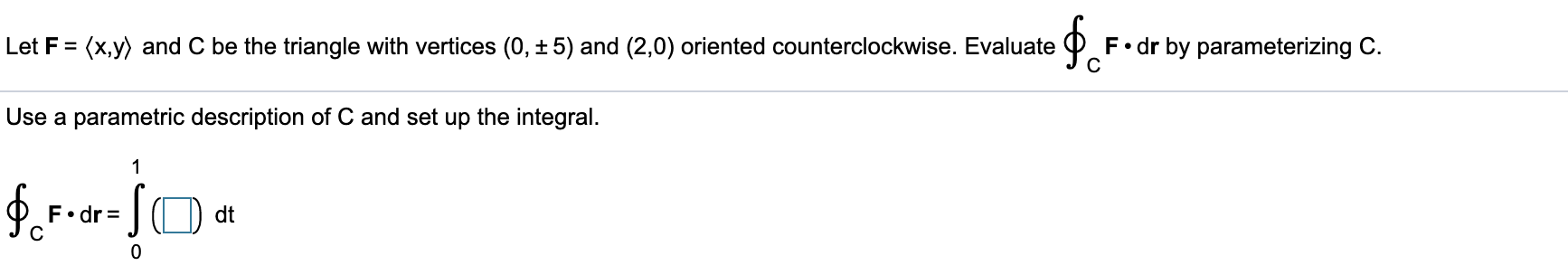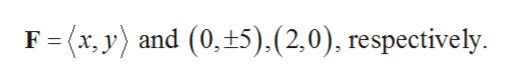F dr by parameterizing CC(x,y) and C be the triangle with vertices (0, ± 5) and (2,0) oriented counterclockwise. EvaluateLet FUse a parametric description of C and set up the integral.1dtF.drC0

Question

How do you set up the integral?help_outlineImage TranscriptioncloseF dr by parameterizing C C (x,y) and C be the triangle with vertices (0, ± 5) and (2,0) oriented counterclockwise. Evaluate Let F Use a parametric description of C and set up the integral. 1 dt F.dr C 0 fullscreen
Step 1

Given a triangle with function and the vertices,help_outlineImage TranscriptioncloseF (x, y) and (0, 5).(2,0), respectively. fullscreen
Step 2

Draw a triangle with the given vertices and mark the parametrization as shown in below figure.

Step 3

Observed from the above figure th...

Want to see the full answer?

See Solution

Want to see this answer and more?

Our solutions are written by experts, many with advanced degrees, and available 24/7

See Solution
Tagged in

Integration